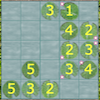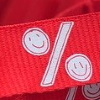# Resources tagged with: Interactivities

Filter by: Content type:
Age range:
Challenge level:

### There are 94 results

Broad Topics > Physical and Digital Manipulatives > Interactivities### More Number Pyramids

##### Age 11 to 14Challenge Level

When number pyramids have a sequence on the bottom layer, some interesting patterns emerge...### Partitioning Revisited

##### Age 11 to 14Challenge Level

We can show that (x + 1)² = x² + 2x + 1 by considering the area of an (x + 1) by (x + 1) square. Show in a similar way that (x + 2)² = x² + 4x + 4### Picturing Triangular Numbers

##### Age 11 to 14Challenge Level

Triangular numbers can be represented by a triangular array of squares. What do you notice about the sum of identical triangle numbers?### Excel Interactive Resource: Fraction Multiplication

##### Age 11 to 16Challenge Level

Use Excel to explore multiplication of fractions.### Power Crazy

##### Age 11 to 14Challenge Level

What can you say about the values of n that make $7^n + 3^n$ a multiple of 10? Are there other pairs of integers between 1 and 10 which have similar properties?### Excel Interactive Resource: Interactive Division

##### Age 11 to 16Challenge Level

Use an Excel to investigate division. Explore the relationships between the process elements using an interactive spreadsheet.### Attractive Tablecloths

##### Age 14 to 16Challenge Level

Charlie likes tablecloths that use as many colours as possible, but insists that his tablecloths have some symmetry. Can you work out how many colours he needs for different tablecloth designs?### Disappearing Square

##### Age 11 to 14Challenge Level

Do you know how to find the area of a triangle? You can count the squares. What happens if we turn the triangle on end? Press the button and see. Try counting the number of units in the triangle now. . . .### Got It

##### Age 7 to 14Challenge Level

A game for two people, or play online. Given a target number, say 23, and a range of numbers to choose from, say 1-4, players take it in turns to add to the running total to hit their target.### Jam

##### Age 14 to 16Challenge Level

To avoid losing think of another very well known game where the patterns of play are similar.### Sliding Puzzle

##### Age 11 to 16Challenge Level

The aim of the game is to slide the green square from the top right hand corner to the bottom left hand corner in the least number of moves.### Guesswork

##### Age 14 to 16Challenge Level

Ask a friend to choose a number between 1 and 63. By identifying which of the six cards contains the number they are thinking of it is easy to tell them what the number is.### The Triangle Game

##### Age 11 to 16Challenge Level

Can you discover whether this is a fair game?### Cogs

##### Age 11 to 14Challenge Level

A and B are two interlocking cogwheels having p teeth and q teeth respectively. One tooth on B is painted red. Find the values of p and q for which the red tooth on B contacts every gap on the. . . .### Number Pyramids

##### Age 11 to 14Challenge Level

Try entering different sets of numbers in the number pyramids. How does the total at the top change?### Changing Places

##### Age 14 to 16Challenge Level

Place a red counter in the top left corner of a 4x4 array, which is covered by 14 other smaller counters, leaving a gap in the bottom right hand corner (HOME). What is the smallest number of moves. . . .### Excel Interactive Resource: Number Grid Functions

##### Age 11 to 16Challenge Level

Use Excel to investigate the effect of translations around a number grid.### Excel Interactive Resource: Equivalent Fraction Bars

##### Age 11 to 16Challenge Level

A simple file for the Interactive whiteboard or PC screen, demonstrating equivalent fractions.### Excel Interactive Resource: Long Multiplication

##### Age 11 to 16Challenge Level

Use an Excel spreadsheet to explore long multiplication.### Excel Interactive Resource: Multiples Chain

##### Age 11 to 16Challenge Level

Use an interactive Excel spreadsheet to investigate factors and multiples.### More Magic Potting Sheds

##### Age 11 to 14Challenge Level

The number of plants in Mr McGregor's magic potting shed increases overnight. He'd like to put the same number of plants in each of his gardens, planting one garden each day. How can he do it?### Have You Got It?

##### Age 11 to 14Challenge Level

Can you explain the strategy for winning this game with any target?### Excel Interactive Resource: Fraction Addition & Fraction Subtraction

##### Age 11 to 16Challenge Level

Use Excel to practise adding and subtracting fractions.### Excel Interactive Resource: Haitch

##### Age 11 to 16Challenge Level

An Excel spreadsheet with an investigation.### Excel Interactive Resource: the up and Down Game

##### Age 11 to 16Challenge Level

Use an interactive Excel spreadsheet to explore number in this exciting game!### Interactive Number Patterns

##### Age 14 to 16Challenge Level

How good are you at finding the formula for a number pattern ?### Countdown Fractions

##### Age 11 to 16Challenge Level

Here is a chance to play a fractions version of the classic Countdown Game.### Matter of Scale

##### Age 14 to 16Challenge Level

Prove Pythagoras' Theorem using enlargements and scale factors.### Latin Lilies

##### Age 7 to 14Challenge Level

In this game you are challenged to gain more columns of lily pads than your opponent.### A Tilted Square

##### Age 14 to 16Challenge Level

The opposite vertices of a square have coordinates (a,b) and (c,d). What are the coordinates of the other vertices?### Estimating Angles

##### Age 7 to 14Challenge Level

How good are you at estimating angles?### Overlap

##### Age 11 to 14Challenge Level

A red square and a blue square overlap so that the corner of the red square rests on the centre of the blue square. Show that, whatever the orientation of the red square, it covers a quarter of the. . . .### Colour in the Square

##### Age 7 to 16Challenge Level

Can you put the 25 coloured tiles into the 5 x 5 square so that no column, no row and no diagonal line have tiles of the same colour in them?### Drips

##### Age 7 to 14Challenge Level

An animation that helps you understand the game of Nim.##### Age 14 to 16Challenge Level

A counter is placed in the bottom right hand corner of a grid. You toss a coin and move the star according to the following rules: ... What is the probability that you end up in the top left-hand. . . .### Proof Sorter - Quadratic Equation

##### Age 14 to 18Challenge Level

This is an interactivity in which you have to sort the steps in the completion of the square into the correct order to prove the formula for the solutions of quadratic equations.### The Bridges of Konigsberg

##### Age 11 to 18Challenge Level

Investigate how networks can be used to solve a problem for the 18th Century inhabitants of Konigsberg.### Tilted Squares

##### Age 11 to 14Challenge Level

It's easy to work out the areas of most squares that we meet, but what if they were tilted?### Square It

##### Age 11 to 16Challenge Level

Players take it in turns to choose a dot on the grid. The winner is the first to have four dots that can be joined to form a square.### Inside Out

##### Age 14 to 16Challenge Level

There are 27 small cubes in a 3 x 3 x 3 cube, 54 faces being visible at any one time. Is it possible to reorganise these cubes so that by dipping the large cube into a pot of paint three times you. . . .### Tilting Triangles

##### Age 14 to 16Challenge Level

A right-angled isosceles triangle is rotated about the centre point of a square. What can you say about the area of the part of the square covered by the triangle as it rotates?### Treasure Hunt

##### Age 7 to 14Challenge Level

Can you find a reliable strategy for choosing coordinates that will locate the treasure in the minimum number of guesses?### Magic Potting Sheds

##### Age 11 to 14Challenge Level

Mr McGregor has a magic potting shed. Overnight, the number of plants in it doubles. He'd like to put the same number of plants in each of three gardens, planting one garden each day. Can he do it?### Magic W

##### Age 14 to 16Challenge Level

Find all the ways of placing the numbers 1 to 9 on a W shape, with 3 numbers on each leg, so that each set of 3 numbers has the same total.### Got it Article

##### Age 7 to 14

This article gives you a few ideas for understanding the Got It! game and how you might find a winning strategy.### Square Coordinates

##### Age 11 to 14Challenge Level

A tilted square is a square with no horizontal sides. Can you devise a general instruction for the construction of a square when you are given just one of its sides?### Just Rolling Round

##### Age 14 to 16Challenge Level

P is a point on the circumference of a circle radius r which rolls, without slipping, inside a circle of radius 2r. What is the locus of P?### Cushion Ball Interactivity

##### Age 14 to 16Challenge Level

The interactive diagram has two labelled points, A and B. It is designed to be used with the problem "Cushion Ball"### Fractions and Percentages Card Game

##### Age 11 to 16Challenge Level

Can you find the pairs that represent the same amount of money?### Spot the Card

##### Age 14 to 16Challenge Level

It is possible to identify a particular card out of a pack of 15 with the use of some mathematical reasoning. What is this reasoning and can it be applied to other numbers of cards?